﻿ The Problem of Forming and Developing Students’ Logical Thinking in the Context of Subject Specialization in Secondary School

### The Problem of Forming and Developing Students’ Logical Thinking in the Context of Subject Specializ...

N. A.Tarasenkova, I. A. AkulenkoOPEN ACCESSPEER-REVIEWED

## The Problem of Forming and Developing Students’ Logical Thinking in the Context of Subject Specialization in Secondary School

N. A.Tarasenkova1,, I. A. Akulenko1

1Bohdan Khmelnytsky National University at Cherkasy, Cherkasy, Ukraine

### Abstract

The article considers the certain features of the development of learners’ logical reasoning in classes with different subject-specialization at secondary school. A short author’s review of literature suggests that three main directions have been formed in the theory of teaching mathematics in the last 50-60 years in . The author investigates them in brief. The analysis shows that the current approach to forming pupils’ logical thinking, significantly varies depending on the subject-specialization and the level of mathematics training. More detailed investigation of particular methods and techniques of teaching the elements of mathematical logic in classes with advanced study of mathematics are given in the article.

### At a glance: Figures

1
Prev Next

• A.Tarasenkova, N., and I. A. Akulenko. "The Problem of Forming and Developing Students’ Logical Thinking in the Context of Subject Specialization in Secondary School." American Journal of Educational Research 2.12B (2014): 33-40.
• A.Tarasenkova, N. , & Akulenko, I. A. (2014). The Problem of Forming and Developing Students’ Logical Thinking in the Context of Subject Specialization in Secondary School. American Journal of Educational Research, 2(12B), 33-40.
• A.Tarasenkova, N., and I. A. Akulenko. "The Problem of Forming and Developing Students’ Logical Thinking in the Context of Subject Specialization in Secondary School." American Journal of Educational Research 2, no. 12B (2014): 33-40.

 Import into BibTeX Import into EndNote Import into RefMan Import into RefWorks

### 1. Introduction

Modernization of education system in Ukraine nowadays is realized through subject-specialization at complete secondary school [24, 25]. The following ideas form the basis of mathematical preparation of senior students during school training: mathematical preparation is carried out in several directions and few levels (Standard Level, Academic Level, Subject-specialised Level and Level of Advanced Mathematics Study  (2003), or Standard Level and Level of Advanced Mathematics Study  (2013)) but only after the pupils receive a general base of education and decide on the further choice; one should make free choice of the direction and level of math training and the school should provide as many directions of training as possible; the mathematics is included to the list of basic subjects in any direction (physical and mathematical, technical, humanitarian); realization of subject-specialized training of mathematics should be carried out in terms of its objectives, its features of the content and form in comparison with training mathematics in general educational classes.

The analysis of mathematics teaching objectives in classes of different directions allows us to identify core goals common to all of them. One such purpose, for example, is an intellectual personality development, especially the development of students' logical thinking. Logical and coherent way of thinking is necessary for pupils of different directions as it is essential for investigating phenomena of various origin (social, humanitarian, polytechnic, natural) and contributes to the development of personal qualities, critical attitude towards oneself and the environment, and because it helps to build pupil’s ability to produce a wide range of hypotheses, innovative ideas and solutions of problems based on identification of the core elements of the studied phenomenon. Therefore, the development of students’ logical reasoning has always been in the center of attention of both theory and practice of mathematics teaching.

The purpose of this paper is to investigate the features of the development of learners’ logical reasoning in classes of different directions with more detailed focus on particular aspects of student learning of mathematical logic elements in classes with Advanced Mathematics Study Level.

### 2. Methodology of Research

To achieve the objectives we will consider four directions each requiring corresponding level of mathematics instruction. Firstly, “social and humanitarian” direction requires mathematics instruction at a standard level. Education Content is directed at the students’ perception of mathematics as a component of general culture. Secondly, “natural sciences” direction prescribes mathematics instruction at academic level and focuses on applied aspects of mathematics. It is supposed for those cases, when mathematics is closely connected with the subject-specialized disciplines and assists in their effective mastering and also for those senior students who have not made up their mind yet concerning the sphere of their future specialization. Thirdly, “physics-mathematical” direction considers mathematics to be taught on Subject-specialised Level with a focus on abstract mathematical concepts. In this case mastering mathematics is oriented to the future students’ profession which is closely connected with mathematics or its practical application. Finally, “pure mathematical” direction in which math is explored in depth level.

2.1. Literature Review

Our review of literature suggests that in the theory of teaching mathematics in the last 50-60 years, three main directions have been formed. The first direction is to introduce a special course “Logic” in secondary school. This direction was supported by A. Ivin , V. Sereda , I. Khomenko , V. Gladunsky , etc. According to these authors, the study of set theory and mathematical logic as a separate course creates a unified methodological basis for mastering different disciplines such as social, humanitarian, natural and mathematical sciences.

The second direction is represented in the works of the authors, who consider the problem of including a minimum amount of classical formal logic concepts in the regular course for their special study (A. Stolyar , I. Nikholskaya , K. Malaniuk , T. Malikov  etc.). The specifics of such work in secondary school is pointed out by I. Nikolskaya , for grades 1-5 by K. Malaniuk , for grades 7-8 by - L. Latotin , while A. Stolyar  and A. Markushevich  consider the problem of using elements of mathematical logic to streamline and improve the logical construction of the school mathematics course. In particular, A. Stolyar  works out the questions of reasoning as to the gradual development of culture in the process of mathematics teaching in primary, middle and secondary school through the logical structuring the empirical data and by using the elements of logical-mathematical language as tools for special type of visibility: “logical visibility”. He also considers the use of formal logic concepts while studying advanced mathematics in elective classes.

A. Kuzhel  stresses the logical foundations of proper construction of school mathematics course in several papers. I. Nicholskaya  considers the problem of reducing logical illiteracy and forming logical culture as a necessary and important part of the general culture of thinking, and investigates the theoretical possibility of incorporating elements of logic to modern school mathematics courses.

The third line of research focuses on the development of methodological provision, mediated formation and development of pupils’ logical skills in the process of learning mathematics. It is reflected in the works of N. Tereshin , D. Poya , G. Sarantsev , Z. Slepkan  etc. The dissertation research of A. Grishko  is devoted to the peculiarities of forming the junior pupils’ ability of thinking clearly.

R. Zagoruy  analyzes the process of forming the specific children’s skills of doing different kinds of reasoning in primary school; M. Shardakov  formulates the requirements for the system of tasks aimed at developing the ability of making inductive inferences. We propose the method of forming the special differentiated system of exercises with an increased logical capacity that serves to develop the junior pupils’ logical thinking.

In the practice of mathematics teaching, one can witness the whole range of approaches: from a lot of efforts to implement actively the elements of logic, together with the appropriate symbols and terminology in school mathematics in 1960’s to the complete abandonment of them in 1980’s. Changing priorities in the education of early 1990’s again pay attention to the need of logical foundations of school mathematics and the methodical support of forming pupils’ specific logic knowledge and skills.

2.2. Practical Implementation of Theoretical Research

Now the results of theoretical and practical research on the problem, made during previous years, actively put into math training practice at primary, secondary and comprehensive high school. A special course on the choice “Logic” is offered for pupils of primary schools, the section “Elements of Mathematical Logic” is included to the program and mathematics textbook in advanced mathematic classes .

### 3. Discussion and Results

3.1. Elements of Mathematical Logic in Classes with Different Level of Mathematics Training

Analysis shows that the methodology of forming pupils’ logical thinking, significantly varies depending on the direction and level of mathematics training (Table 1).

3.2. Elements of Mathematical Logic in Classes with Advanced Study of Mathematics

For more detail we will focus on the features of methods and techniques of teaching students the elements of mathematical logic in classes with advanced study of mathematics.

The program of mathematics for schools and classes with advanced study points out that the students, who master it on the advanced level should be focused on further activities in the development of mathematical science, and, therefore, should have skills in operating mathematical logic. So, at the beginning of the 10th grade, they study the theme “Elements of Mathematical Logic”, designed for introducing the relevant apparatus of mathematical logic.

There are some methodological approaches for teaching students the elements of mathematical logic. One of them proposes the introduction of key concepts on the basis of deductive scheme, beginning with more precise definitions of key concepts. In our opinion, such approach is methodically substantiated in higher school. The study of mathematical logic theory elements school should be motivated by considering the examples of problems with solution based on the use of so-called “common sense”.

#### Table 1. Guidelines of the Formation and Development of Pupils’ Logical Thinking

In the process of solving such problems students demonstrate the ability to think sequentially, to refute wrong conclusions, to select relevant and valid arguments. These capabilities require further involving, improving and developing both actual mathematical skills and social experience of students. To achieve this goal, the so-called problem of “knights and liars”, the task to establish a correspondence between objects, “the problem to the truth” (when it is required to establish the truth or falsity of one or more statements) can be used.

For example, firstly, one can offer the students the following problems.

Objective 1. Imagine that you are in the room that has two doors. One door points the way to freedom, and the other – to an imminent death. There is one guard near each of the doors. One of the guards tells only the truth, the other only lies. Guards can answer only “yes” or “no”. You don’t know where the door to freedom is and which of the guards stands at that door. However, you know that the guards are aware of the point, who of them is the liar and who tells the truth. You can ask only one question and only to one of the guards in order to find the correct door which leads to freedom. What question should you ask?

Objective 2 (“Three Friends”). Three school graduate friends Igor, Ivan and Oleg were going to enter colleges in different cities of Ukraine: Donetsk, Kiev and Kharkov. A graduate of the class with advanced study of mathematics tried to enter a college in Donetsk, but failed. A graduate of the class with advanced study of chemistry entered Kharkov University. Oleg confirmed his deep knowledge of jurisprudence during the interview, which he had received in the class of the corresponding profile and entered the faculty of law. Ivan refused to accept the parents’ advice to study in Kharkiv. In which city did the boys continue their studies?

In the process of solving these problems it is advisable to emphasize that students have used the logical laws intuitively, but these laws can be represented verbally (Table 2) or with the help of symbols.

However, it should be pointed out that intuitive knowledge may not always be sufficient to reach a conclusion on the validity of reasoning. For example, the students may be asked to determine if the conclusion below is valid (objective 3).

Objective 3. Determine if the conclusion below are true: “If a quadrangle happens to be a rhombus, then its diagonals are mutually perpendicular. If the diagonals of a quadrangle are not mutually perpendicular, then the quadrangle cannot be a square. If a quadrilateral is a square, then it can be fitted into a circle. What is not true means that a given quadrangle cannot be fitted into a circle or that its diagonals are not mutually perpendicular. As a result, the quadrangle is neither a rhombus, nor a square”.

Trying to give a reasonable answer to this question may cause some difficulties. It proves the need for instruction in the field of mathematics that allows to “calculate reasoning”, without being bound to the content. Students are encouraged to seek historical information related to the inception and development of mathematical logic, outstanding mathematicians that have contributed to this field of science and it’s current and relevant problems.

Next, we provide a list of key concepts that, according to the program in mathematics for schools and classes with advanced study, the students should be able to define and describe:

•  statements and logical operations applicable to them;

•  predicates, and logical operations applicable to them;

•  equivalent statements and equivalent predicates;

•  truth functions;

•  domain and range of the truth predicates;

•  quantifiers, both existential and universal;

•  formulas of Boolean Algebra;

•  formulas of predicate calculus.

Let’s now discuss distinct features that characterize how these concepts may be introduced.

Introducing the concept of statement, it is advisable to carry out the following concrete inductive scheme (in the process of practicing exercises), pointing out the characteristic features of this concept, giving examples and counter examples, practicing exercises with the purpose of leading the object to the concept and making conclusions of belonging of the object to this concept.

Introducing the concept of a “truth function” is possible through the exercises such as the ones we list below.

Exercise 1. The set of true statements is pointed by symbol T (from English word, True), and the set of false statements is pointed by symbol F (from English word, False). 1) Give the examples of the elements of the sets T and F; 2) which of the claims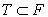,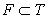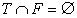is true?

If the statement A is true, it is pointed as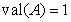or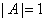. And in this case it is said that A has the meaning of truth 1. If the statement A is False then it is said that A has the meaning of truth 0. In other words, a function can be defined with the range of meanings {0, 1} on a set of given statements. This function is called the function of truth.

Exercise 2. Determine the truth value of statements:

1) the equality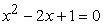is true if and only if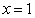;

2) the equality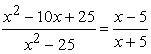is true for all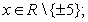3) the equality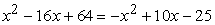is false for any value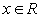;

4) the inequality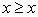is proved for any values;

5) the equality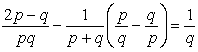is true for all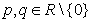.

We introduce the concept of predicate on concrete inductive basis, proposing to determine which of the items 1) - 7) are statements:

1)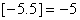; 2)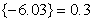; 3)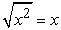; 4)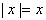; 5)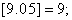6)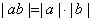; 7)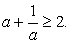We define characteristic features of sentences which are not statements. Thus, the truth value of 3) and 4) depends on the variable x. Item 6) is true for all a and b. Item 7) is true for all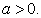We emphasize that if a sentence has a variable, it is necessary to define the domain of this variable. Another specific feature of items 3, 4, 6 and 7 is that if a variable takes a value, then the sentence becomes a statement.

Together with pupils we list characteristic properties of predicates: 1) expression should contain a variable; 2) the domain of the variable must be defined; 3) if a variable takes a value, then an expression becomes a statement. We give the following examples of predicates: 1) on the set of triangles x: the square of one side of the triangle equals the sum of squares of the other two sides; 2) on the set of integers: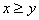; 3) on the set of natural numbers: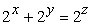.

After summing up, we point out that arbitrary numerical equality or inequality is the example of statement, arbitrary equation or inequality with variables is the example of predicate.

Pupils are offered to choose predicates. If the sentence is not a predicate, it should be completed to become a predicate:

•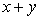(this is not a predicate because properties 2 and 3 are not fulfilled);

•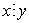(this is not a predicate because the domain is not defined);

•  let’s say M is the set of students, “x practice sports” (predicate);

•  let’s say M is the set of students, “y come to me” (is not a predicate because it is not a statement if y takes a value from M);

•  let M be the set of students, “x and y are sisters” (predicate).

On the basis of the given examples pupils get acquainted with single-variable and two-variable predicates. Special attention should be concentrated on the fact that if one variable of a two-variable predicate takes a value from the domain, we obtain a single-variable predicate. A statement can be considered as a 0-variable predicate.

The following questions could be useful for the generalization of the concept of the domain: 1) Let’s consider number expression: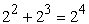. Is it an example of a proposition? Prove your argument. 2) Give an example of single-variable, two-variable, three-variable, four-variable and six-variable predicates. Which of them induce the given proposition when all variable of a predicate take values from the set of natural numbers?

The concept of equivalent predicates is useful to introduce using the technique of analogues with preliminary actualization of students’ knowledge about equivalent equations or inequalities. For example, we could assign exercises (5 and 6).

Еxercise 5.

Choose the equation, which is the consequence of equation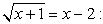A)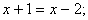B)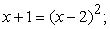C)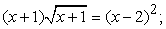D)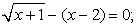E)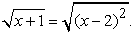Еxercise 6.

Choose the equation, which is equivalent to the equation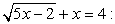A)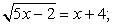B)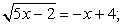C)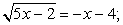D)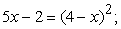E)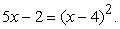It’s useful to remind students of definitions of concepts “equation consequence”, “equivalent equations” in the process of solving these problems. It’s also recommended to remind them which elimination of equations leads to the consequence of equations and which ones to equivalent equations. On this basis, while using analogy, one can introduce the meaning of predicate consequence concept and equivalent predicates. While using the method of comparison and analogy it is possible to give the meaning of operations with predicates.

The method of analogy is beneficial to use for introducing operations with statements. For realizing the meaning of logical operations of conjunction, disjunction, implications, equality of statements, students should be supplied with truth tables of logical operations completed in such a way (Table 3- Table 5).

However, not all the new concepts of the considered theme are to be introduced on concrete-inductive basis. Experience shows that definitions of operations with statements and operations with predicates are better to be introduced following a deductive scheme. This scheme is recommended when universal quantifier and existential quantifier are introduced.

Teachers should pay special attention for reviling the logical structure of mathematical statements and theorems. “Elements of mathematical logic” for pupils who study advanced mathematics in this context is very significant. It should be noted that mathematical facts can be formulated as either categorical or implication statements. Students are proposed to provide examples of theorems formulated with either categorical or implication statements. Then students are recommended to re-formulate theorems formulated with categorical statements using implication statements and vice versa.

To it is necessary to remember while formalizing the formulae of theorem that four types of categorical statements should be chosen (affirmative, partial affirmative, negative, partial negative. It is suggested to use Table 6 for the demonstration of examples of matching symbols and means of formulization. It is advisable to start with examples followed by illustrations using the Euler-Venn diagrams while working with Table 6. The generalized formulation of a certain kind of categorical statements should be considered then and the way they are formalized.

#### Table 6. Four Types of Categorical Statements

It should be recalled that the record of theorems in the form of implicative statements has the structure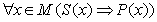in which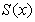is the condition of the theorem,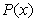is a conclusion. Explanatory part of the theorem contains a list of sets M, on which the predicatesare specified. The explanatory part of the theorem is often omitted for shortening formulations. Students are suggested to create all possible types of implicative statements and matching theorems on the basis of predicates (Table 7).

#### Table 7. Four types of theorems

Students can be suggested to prove the equivalence of the direct theorem, and the opposite of the inverse one. They can do it in the following way. Since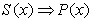-is a direct theorem, then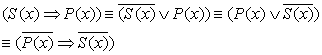. The system of exercises directed on pupils’ mastering of mathematical statement formulation should provide performing direct and opposite action. Taking this into consideration, it is useful to complete the following exercises together with pupils.

Exercise 1. Write down a statement with a help of quantifiers and specify their negations.

•  Every single number is an odd number.

•  All natural numbers are whole numbers, but not all whole numbers are natural ones.

•  A set N (natural numbers) contains the smallest number.

•  A set R (real numbers) contains neither the smallest nor the largest number.

•  For the preassigned arbitrary triangle there is a matching triangle equal to it in the specified location on a given ray.

•  For the arbitrary straight line, there are points lying and not lying on it.

•  For any two points on the straight line, there are points lying between them.

Exercise 2. “Move” symbolic record into the native language, determine the validity of each of the statements: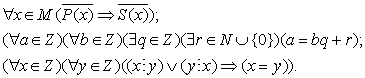Exercise 3. Write down stereometry axioms and some theorems with the help of quantifiers and symbols.

Axiom 1. There are points lying in the given plane, and points not lying there.

Axiom 2. Through 3 arbitrary points that are not lying on the same line, we can draw only one plane.

Axiom 3. If two points of a line lie in a plane, then every point of this line lies in this plane.

Theorem 1. One and only one plain can be drawn through two intersecting lines.

Theorem 2. Only one plain can be drawn via a line and a point not lying on it.

Theorem 3. The diagonals of a rhombus intersect at right angles.

Theorem 4. A line parallel to one of two given parallel lines is parallel to other given line.

3.3. Considering the Applied Aspect of the “Elements of Mathematical Logic”

As it was mentioned in the mathematics curriculum for advanced classes, different approaches should be applied for the mathematical training of students: they should be prepared for obligatory mastering of specific concepts and facts of mathematical curriculum (the theoretical aspects), as well as for using the skills for modeling of real processes, and solving applied tasks. Students should be oriented on systematic use of ideas and methods of mathematics in natural sciences. The applied aspect of the “Elements of mathematical logic” can be realized through the analysis and synthesis of relay-contact or combined circuits. Students are suggested with a problem situation.

Problem situation. At the entrance of a three-story house, there is only one lamp. It is necessary to create the electrical circuit of the house in the way by using one’s own key: a lamp on each floor could be switched on and switched off at the entrance regardless of the position of switchers on other floors.

It is possible to solve the given problem by using elements of the theory of relay contact circuits. It is necessary to point out the idea of relay contact circuit of connecting and disconnecting contact, of function of conductivity matching the relay contacting circuit among the ideas which deserve to be considered.

The consideration of sequent and parallel connections of conductors makes it possible to establish some specific analogy with calculus of statements, to be more exact: the consequence circuit realizes the operation of conjunction, and the parallel - the disjunction operation (Figure 1).

The main task of the relay contact circuit theory is the analysis (building on the given circuit with its matching formula and the function of conductivity) and the synthesis (building of the circuit according to the formula or the function of conductivity). For consolidation of basic ideas the following exercises can be suggested.

Exercise 1. Create the function of conductivity for the ladder circuit (relay contact circuit) given on Figure 2.

The solution can be built this way: the contact A is connected successively with a group of contacts (they are limited by a dotted line), which, in its turn, is the parallel connection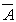or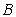. For this reason, the matching formula looks like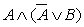. It can be simplified by using equal logical formulas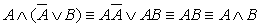.

Now, it is necessary to build the truth table for the last formula to find the matching function of conductivity.

Exercise 2. Simplify relay contact circuit shown on Figure 3.

To solve this problem, it is possible to recommend students the following three stages: 1) to build formula according to the circuit; 2) to simplify the formula (if it is possible); 3) to build a new circuit on simplified formula.

The circuit on Figure 3 matches the formula: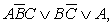can be simplified: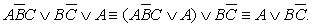The last formula can be used to build the simplified circuit.

### 4. Conclusion

Current instruction practices related to the introduction of elements of mathematical logic in secondary schools, though intense, deliver mixed results. There are a number of approaches for introducing concepts of set theory and mathematical logic in school curricula. However, the curricula do not adequately investigate applied aspects of mathematical logic. This study argues for the inclusion of the applied aspects side by side with the abstract aspects of mathematical logic and set theory in school curricula. The applied aspects of math logic are also worth focusing on in the process of mathematical and methodical training of future Mathematics teachers. Since they form, as our research reveals [26, 27], “problem areas” in students’ mathematical and methodical training and predetermine their beliefs about the nature of Mathematics.

### Acknowledgement

We would like to thank the authors of the references who have helped us indirectly through their books, journals while preparing this manuscript.

### References

  Ivin, A.A.Logic,Artek, Kyiv, 1996, 232.In article  Sereda, V.Yu. Learn to think logically, Rad. shkola, Kyiv, 1989, 175.In article  Hladunsky, V.N. Methodological foundations for the study course of logic in general, specialized and specialized schools, Institute of Pedagogy and Psychology of Professional Education of APS Ukraine, Kyiv, 1998, 20.In article  Khomenko, I.V., Aleksyuk, I.A.Fundamentals of logic, GoldenGate, Kyiv,1996, 256.In article  Stolyar, A.A. Why and how do we prove in mathematics: Conversations with senior pupils, Nar. osvita, Minsk, 1987, 142.In article  Stolyar, A.A. “The role of mathematics in the humanization of education”, Mathematics at school, 6, 5-7, Jul. 1990.In article  Nicholskaya, I.L. Acquaintance with mathematical logic, Moscow psycho-social institution, Flint, Moscow, 1998,128.In article  Nicholskaya, I.L. Instilling logical literacy while teaching mathematics, Moscow, 1973, 185.In article  Malanyuk, E.P. Forming pupils’ (grades 1-5) logical literacy in the process of teaching mathematics, Kyiv, 1979, 24.In article  Malikov, T.S. Inductive and deductive reasoning as a means of development of students’ activity and critical thinking while teaching mathematics, Moscow, 1988, 16.In article  Latotin, L.A. Development of logic thinking of pupils of 4-8 classes through the study of logical operations and relations (in the algebraic material), Minsk, 1982, 17.In article  Markushevich, A.I. “On the immediate tasks of school teaching”, On upgrade paths of school mathematics, Education, Moscow, 1978, 29-48.In article  Kuzhel, A.V. “Logical foundations of school mathematics course” Mathematics at school, 1, 3-6, Jan. 1999, 3, 7-9, Mar. 1999.In article  Poya, D. Mathematics and plausible reasoning, Science, Мoscow, 1975. [E-book] Available: http://www.ega-math.narod.ru/Books/Polya.htm [Accessed Aug. 4, 2014]In article  Sarantsev, G.I. Teaching mathematical proof at school, Education, Мoscow, 2000, 174.In article  Tereshin, N.A. Mathematics teacher’s methodical system in the development of pupils’ scientific outlook, Moscow, 1991, 44.In article  Slepkan, Z.I. Methodical system implementing developmental function of teaching mathematics at school, Moscow, 1987, 47.In article  Grishko, O.I. Formation of senior pupil’s skills to argue convincingly while teaching mathematics, Kyiv. 1994, 199sIn article  Zagoruy, R.V. Senior pupil’s mastering in making conclusions (on materials of Mathematics), Kyiv, 1990, 22.In article  Shardakov, M.N. Thinking of a pupil, Uchpedgiz, Moscow, 1963, 256. [E-book] Available: http://elib.gnpbu.ru/text/shardakov_myshlenie-shkolnika_1963/fs,1/ [Accessed Aug. 4, 2014]In article  Lyman, F.M. Mathematical logic and the theory of algorithms, “Slobozhanzshina”, Sumy, 1998, 152.In article  Shevchenko, V.E. Some ways to solve logical problems, High School, Moscow, 1978, 80.In article  Merzlyak, A.G., Nomirovsky, D.A., Polonsky, V.B., Yakir. M.S, Algebra and analysis beginning: textbook for grade 10 with advanced study of mathematics, Gimnaziya, Kharkiv, 2010, 415.In article  Concept of subject-specialised education at high school, Information anthology of Ministry of education and science of Ukraine, № 24, 3-15, 2003.In article  On Approval of the Concept of subject-specialized education at high school. Order of Ukraine Ministry of education № 1456 10/21/13. Available: http://osvita.ua/legislation/Ser_osv/37784/ [Accessed Aug. 4, 2014]In article  Akulenko I. A. “Clarify the problem of future math teachers’ of specialized schools methodical training”, Science and Education a New Dimension, Budapest, V. 5, 48-53. 2013. Available: http://seanewdim.com/uploads/3/2/1/3/3213611/akulenko_i._to_the_problem_of_investigating_the_profile_ school_teachers_methodical_preparation.pdf [Accessed Aug. 4, 2014]In article  Tarasenkova, N. A., Akulenko, I. A., “Determination of Students’ Beliefs is one of the Aspects of Competence Oriented System of Mathematics Teachers’ Methodical Preparation.” American Journal of Educational Research 1, no. 11, 477-483, 2013. Available: http://pubs.sciepub.com/education/1/11/4/index.html [Accessed Aug. 4, 2014]In article CrossRef